# Line segment

For the line segment whose endpoints are L[-1, 13] and M[18, 2], find the x and y value for the point located 4 over 7 the distance from L to M.

x =  9.86
y =  6.71

### Step-by-step explanation:

$x=-1+4\mathrm{/}7\cdot \left(18+1\right)=9.86$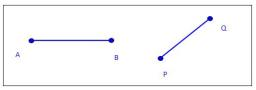Did you find an error or inaccuracy? Feel free to write us. Thank you!Tips to related online calculators
Line slope calculator is helpful for basic calculations in analytic geometry. The coordinates of two points in the plane calculate slope, normal and parametric line equation(s), slope, directional angle, direction vector, the length of the segment, intersections of the coordinate axes, etc.
Check out our ratio calculator.

## Related math problems and questions:

• Points on line segmentPoints P & Q belong to segment AB. If AB=a, AP = 2PQ = 2QB, find the distance: between point A and the midpoint of the segment QB.
• Find the 3Find the distance and midpoint between A(1,2) and B(5,5).
• The coordinates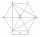The coordinates (5, 2) and (-6, 2) are vertices of a hexagon. Explain how to find the length of the segment formed by these endpoints. How long is the segment?
• Distance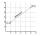Calculate distance between two points X[18; 19] and W[20; 3].
• Midpoint of segmentPoint A has coordinates [4; -11] and the midpoint of the segment AB is the point [17; -7]. What are the coordinates of point B?
• Distance between 2 pointsFind the distance between the points (7, -9), (-1, -9)
• Construct 8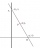Construct an analytical geometry problem where it is asked to find the vertices of a triangle ABC: the vertices of this triangle must be the points A (1,7) B (-5,1) C (5, -11). the said problem should be used the concepts of: distance from a point to a li
• V - slopeThe slope of the line whose equation is -3x -9 = 0 is
• Midpoint 4If the midpoint of a segment is (6,3) and the other endpoint is (8,-4), what is the coordinate of the other end?
• Coordinates of midpointIf the midpoint of the segment is (6,3) and the other end is (8,4) what are the coordinate of the other end?
• Slope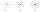Calculate the slope of a line that intersects points (-84,41) and (-76,-32).On a graph, Point Y is located at (4, -2). Point Z is located 5 units to the left of Point Y. In which quadrant is Point Z located?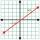What is the slope of the perpendicular bisector of line segment AB if A[9,9] and B[9,-2]?Find the equation of a line given the point X(8, 1) and slope -2.8. Arrange your answer in the form y = ax + b, where a, b are the constants.The 4 cm long line segment is enlarged in the ratio of 5/2. How many centimeters will measure the new line segment?A=(x, x) B=(1,4) Distance AB=√5, find x;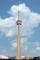A cell tower is located at coordinates (-5, -7) and has a circular range of 12 units. If Mr. XYZ is located at coordinates (4,5), will he be able to get a signal?